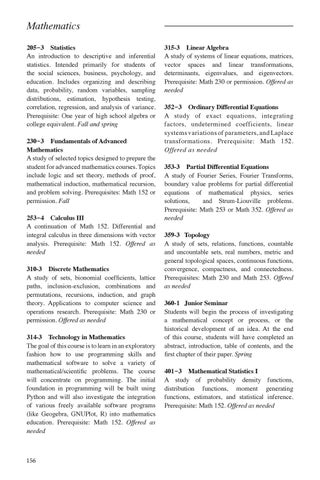Mathematics 205‑3  Statistics An introduction to descriptive and inferential statistics. Intended primarily for students of the social sciences, business, psychology, and education. Includes organizing and describing data, probability, random variables, sampling distributions, estimation, hypothesis testing, correlation, regression, and analysis of variance. Prerequisite: One year of high school algebra or college equivalent. Fall and spring 230‑3  Fundamentals of Advanced Mathematics A study of selected topics designed to prepare the student for advanced mathematics courses. Topics include logic and set theory, methods of proof, mathematical induction, mathematical recursion, and problem solving. Prerequisites: Math 152 or permission. Fall 253‑4  Calculus III A continuation of Math 152. Differential and integral calculus in three dimensions with vector analysis. Prerequisite: Math 152. Offered as needed 310-3  Discrete Mathematics A study of sets, bionomial coefficients, lattice paths, inclusion-exclusion, combinations and permutations, recursions, induction, and graph theory. Applications to computer science and operations research. Prerequisite: Math 230 or permission. Offered as needed 314-3  Technology in Mathematics The goal of this course is to learn in an exploratory fashion how to use programming skills and mathematical software to solve a variety of mathematical/scientific problems. The course will concentrate on programming. The initial foundation in programming will be built using Python and will also investigate the integration of various freely available software programs (like Geogebra, GNUPlot, R) into mathematics education. Prerequisite: Math 152. Offered as needed

156

315-3  Linear Algebra A study of systems of linear equations, matrices, vector spaces and linear transformations, determinants, eigenvalues, and eigenvectors. Prerequisite: Math 230 or permission. Offered as needed 352‑3  Ordinary Differential Equations A study of exact equations, integrating factors, undetermined coefficients, linear systems variations of parameters, and Laplace transformations. Prerequisite: Math 152. Offered as needed 353-3  Partial Differential Equations A study of Fourier Series, Fourier Transforms, boundary value problems for partial differential equations of mathematical physics, series solutions, and Strum-Liouville problems. Prerequisite: Math 253 or Math 352. Offered as needed 359-3 Topology A study of sets, relations, functions, countable and uncountable sets, real numbers, metric and general topological spaces, continuous functions, convergence, compactness, and connectedness. Prerequisites: Math 230 and Math 253. Offered as needed 360-1 Junior Seminar Students will begin the process of investigating a mathematical concept or process, or the historical development of an idea. At the end of this course, students will have completed an abstract, introduction, table of contents, and the first chapter of their paper. Spring 401‑3  Mathematical Statistics I A study of probability density functions, distribution functions, moment generating functions, estimators, and statistical inference. Prerequisite: Math 152. Offered as needed